# Stirling's approximation in Clojure

November 24, 2010 at 11:12 PM

In my free time, I've been working through Probability Theory: A Concise Course by Y.A. Rozanov. The first chapter contains a great factorial approximation that anyone doing computer-based statistics or combinatorics ought to know: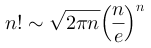This is Stirling's Approximation. As n grows, the relative error of this function compared to n! gets smaller and smaller. More formally, the symbol expresses asypmtotic equivalence, meaning that when A(n) ∼ B(n),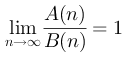I was curious about the actual behavior of this formula, so I decided to plot the expected convergence. To do this, I wrote up simple Clojure versions of both functions and charted the expected convergence using Incanter.

The graph produced by this doesn't exactly pass muster.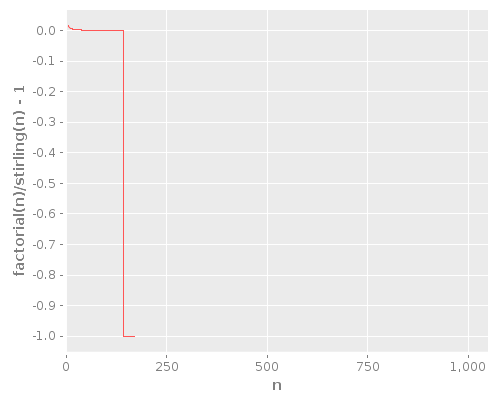We've been bit by one of the classic problems of numerical computing: the way that a number is represented internally limits what can be done with it. In our case, we've exceeded the range of values that a Java Double can handle. It has 8 bytes split into a 52-bit mantissa, an 11-bit exponent, and a single sign bit (in detail). The max value it can hold is 1.7976931348623157×10308. Once we exceed that value, everything is simply "Infinity".

Clojure magically moves from Integer arithmetic to BigInteger arithmetic when we overstep the Integer upper bound of 231-1. But we get no such love from the functions in java.lang.Math.*, which quickly (at n=144) overflow to Infinity.

Now, the entire point of this function is to have a fast approximation of the factorial for large n, so this is an issue that we need to overcome. The naïve version is actually completely useless, since it only functions for small n.

So let's fix it.

We need to convert our function to use an arbitrary precision decimal type. As far as I can tell, Math.PI only comes in double precision. We'd need to have an n of, oh, 9,223,372,036,854,775,807 before we exceeded the Double range and needed to change the way we compute first expression. I don't think anyone is actually going to seriously try doing factorials that large, but if you are, you can make use of this free BigSquareRoot class that uses BigDecimals.

For my purposes, only the exponentiation, division, and multiplication in the second expression need to be converted. Java has a handy BigDecimal class that fits the arbitrary-precision bill:

We also need to use BigDecimal division in the comparison function, but I'll leave that as an exercise for the reader. The outcome is quite pleasing from the numerical error standpoint: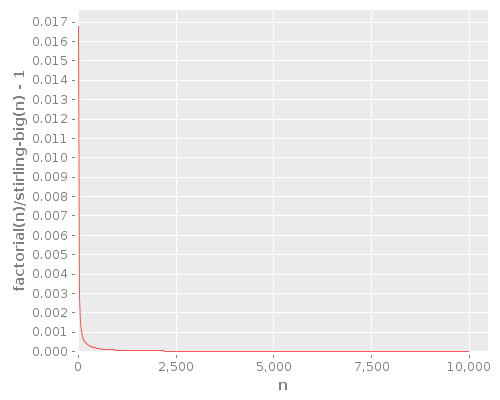You can see that as n gets larger, the ratio of the functions asymptotically approaches 1. The relative error of Stirling's approximation shrinks with larger n, which is great for most applications. The absolute error grows, but the rate at which it grows is smaller than the rate at which the actual value grows.

And the performance is great, too! Check out the differences: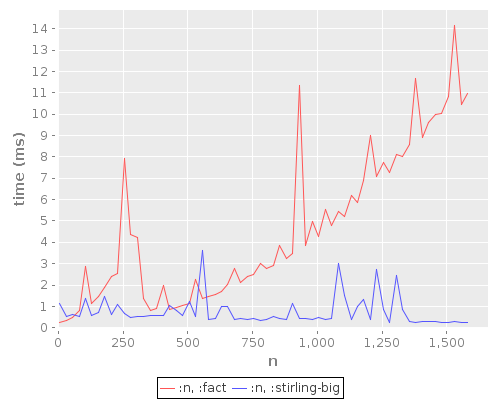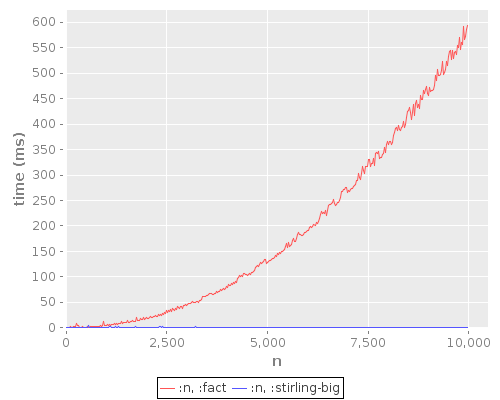The raw factorial function is superior for small n, which is to be expected. The factorial function has noticeable performance perturbations in the local view, but these mostly disappear in the full view. The factorial function seems to perform in quadratic time relative to n, while Stirling's formula has a very low constant cost.

So remember Stirling's formula if you find yourself computing large factorials.

Updated 2010-11-25 07:48 to clarify relative error versus actual value. Thanks to David Karapetyan for pointing out the discrepency.